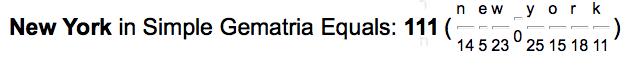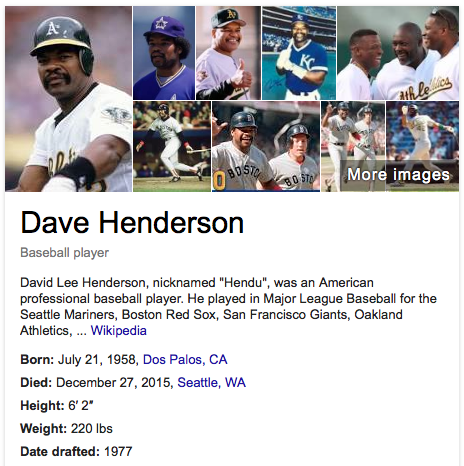## Sunday, December 27, 2015

### 57 86 118 | Dave Henderson of '86 World Series, Dead 57-Days After 111th World Series

David Lee Henderson, best remembered for his '86 heroics in the ALCS to advance to the World Series against the Mets, has died at age 57, 57-days after the Mets lost the 111th World Series, November 1, 2015.  It should be noted that the Mets lost the World Series this year to the Kansas City Royals, who Dave Henderson finished his career with.111th Game of World Series, Played in New York, on 11/1
David = 4+1+4+9+4 = 22 (Dave = 4+1+4+5 = 14)
Lee = 3+5+5 = 13
Henderson = 8+5+5+4+5+9+1+6+5 = 48/57 (World Series = 57, dead 57-days after World Series)
David Lee Henderson = 83/92
Dave Henderson = 62/71

World 5+6+9+3+4 = 27
Series = 1+5+9+9+5+1 = 30/39/48
World Series = 57/66/75

Dave Henderson's 1986 World Series was played between the New York Mets and Boston Red Sox.  The Mets played at Shea, the Red Sox still play at Fenway.

Fenway = 6+5+14+23+1+25 = 74
Shea = 19+8+5+1 = 33

Dave Henderson, who played the majority of his games at Fenway, has died on a date with '74' numerology, a very important number to the Freemasons, who run the MLB, and all of professional sports.

12/27/2015 = 12+27+20+15 = 74
Masonic = 13+1+19+15+14+9+3 = 74

Dave also died in Seattle, on the 47th Parallel, on a date with a life lesson number of '47'.

12/27/2015 = 12+27+(2+0+1+5) = 47
Seattle, on 47th Parallel
Space Needle's restaurant rotating does full rotation every 47 minutes
Time = 20+9+13+5 = 47

As for the year '86, it was most definitely a symbolic moment in Red Sox history.

Symbol = 19+25+13+2+15+12 = 86
Triangle = 20+18+9+1+14+7+12+5 = 86
Pyramid = 16+25+18+1+13+9+4 = 86
**Red Sox didn't win a World Series for 86-years...

Dave's '86 heroics in Game 5 of the ALCS came on the date of October 12, 1986.

10/12/1986 = 10+12+19+86 = 127
10/12/1986 = 10+12+(1+9+8+6) = 46
10/12/1986 = 1+0+1+2+1+9+8+6 = 28
10/12/86 = 10+12+86 = 118 (Death = 118, Jewish Gematria)

It should be noted that Dave Henderson died 38-years after being drafted into Major League Baseball, in 1977, with the 26th pick by the Seattle Mariners, home of the (206).

The game of the memorable hit was played in Anaheim, on the 33rd Parallel, in Orange County.

Anaheim = 1+5+1+8+5+9+4 = 33
Orange = 6+9+1+5+7+5 = 33

Dave Henderson was also the leading offensive player in Game 5 of the World Series, which came on a date with very 'symbolic' numerology, especially in New York.

10/23/1986 = 10+23+19+86 = 138
10/23/1986 = 10+23+(1+9+8+6) = 57
10/23/1986 = 1+0+2+3+1+9+8+6 = 30
10/23/86 = 10+23+86 = 119

All Seeing Eye = 1+12+12+19+5+5+9+14+7+5+25+5 = 119
Star of David = 19+20+1+18+15+6+4+1+22+9+4 = 119
Think about the date September 11 in New York, written 11/9 in most parts of the world.

Notice the name of the President of the Red Sox, more masonic numbers.

Sam = 1+1+4 = 6/15
Kennedy = 2+5+5+5+5+4+7 = 33
Sam Kennedy = 39/48

Sam = 19+1+13 = 33
Kennedy = 11+5+14+14+5+4+25 = 78
Sam Kennedy = 111

http://www.cnn.com/2015/12/27/us/dave-henderson-dies/index.html

Dave Henderson was born July 21, 1958, in Dos Palos, CA, at least supposedly.

7/21/1958 = 7+21+19+58 = 105
7/21/1958 = 7+21+(1+9+5+8) = 51
7/21/1958 = 7+2+1+1+9+5+8 = 33 (Dos Palos, CA = 4+6+1+7+1+3+6+1+3+1 = 33/42)
7/21/58 = 7+21+58 = 86

Notice below, that Dave Henderson wore the #42 in high school, and with the Athletics, matching the gematria of 'Dos Palos', and a few other things...

He died in Seattle, where he began his MLB career.End = 5+5+4 = 14Henderson and I share the same birthday, July 21.  This coming July 21, has a fateful date numerology. 7/21/16 = 7+21+16 = 44 (Kill = 11+9+12+12 = 44)